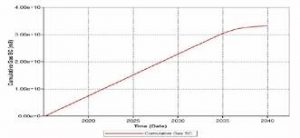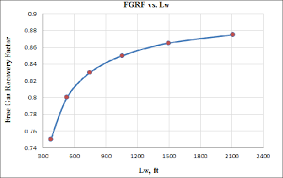## How to Calculate and Solve for Gas Recovery Factor (Production) | Reservoir Fluid FlowThe image above represents a gas recovery factor (G).

To compute for a gas recovery factor (production), two essential parameters are needed and these parameters are cumulative gas production (GP) and initial gas in place (G).

The formula for calculating the gas recovery factor (G):

RFG = Gp / G

Where:

RFG = Gas Recovery Factor (G)
GP = Cumulative Gas Production
G = Initial Gas in Place

Let’s solve an example;
Find the gas recovery factor (G) when the cumulative gas production is 13 and the initial gas in place is 17.

This implies that;

GP = Cumulative Gas Production = 13
G = Initial Gas in Place = 17

RFG = Gp / G
RFG = 13 / 17
RFG = 0.764

Therefore, the gas recovery factor (G) is 0.764.

Calculating the Cumulative Gas Production when the Gas Recovery Factor (G) and the Initial Gas in Place is Given.

GP = RFG x G

Where;

GP = Cumulative Gas Production
RFG = Gas Recovery Factor (G)
G = Initial Gas in Place

Let’s solve an example;
Find the cumulative gas production when the gas recovery factor (G) is 24 and the initial gas in place is 6.

This implies that;

RFG = Gas Recovery Factor (G) = 24
G = Initial Gas in Place = 6

GP = RFG x G
GP = 24 x 6
GP = 144

Therefore, the cumulative gas production is 144.

## How to Calculate and Solve for Gas Recovery Factor of a Reservoir Fluid Flow | The Calculator EncyclopediaThe image above represents a gas recovery factor.

To compute for the gas recovery factor, three essential parameters are needed and these parameters are initial pressure(Pi), initial compressibility factor (Ziand P/Z Ratio (P/Z).

The formula for calculating the gas recovery factor:

RFG = 1 – P/Z.Pi / Zi

Where;

RFG = Gas Recovery Factor (P, Z)
Pi = Initial Pressure
Zi = Initial Compressibility Factor
P/Z = P/Z Ratio

Let’s solve an example;
Find the gas recovery factor when the initial pressure is 12, the initial compressibility factor is 7 and the P/Z ratio is 36.

This implies that;

Pi = Initial Pressure = 12
Zi = Initial Compressibility Factor = 7
P/Z = P/Z Ratio = 36

RFG = 1 – P / Z.Pi / Zi
RFG = 1 – 36[12 / 7]
RFG = 1 – 36[1.714]
RFG = 1 – 61.714
RFG = -60.714

Therefore, the gas recovery factor is -60.714.